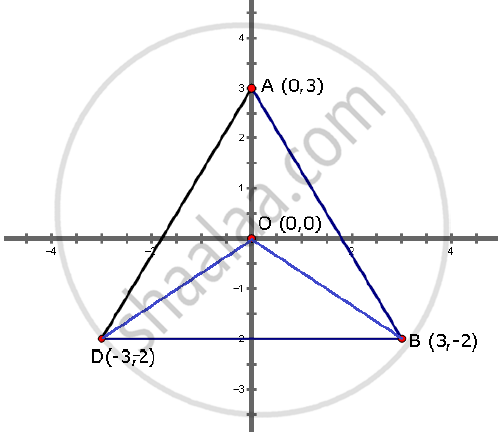Share

Books Shortlist

# Use Graph Paper for this Question) (4) A(0, 3), B(3, −2) and O(0, 0) Are the Vertices of Triangle Abo. (1) Plot the Triangle on a Graph Sheet Taking 2 Cm = 1 Unit on Both the Axes.1 (2) Plot D the Reflection of B in the Y-axis, and Write Its Co-ordinates. (3) Give the Geometrical Name of the Figure Abod. (4) Write the Equation of the Line of Symmetry of the Figure Abod. - ICSE Class 10 - Mathematics

#### Question

Use graph paper for this question) (4)
A(0, 3), B(3, −2) and O(0, 0) are the vertices of triangle ABO.

(1) Plot the triangle on a graph sheet taking 2 cm = 1 unit on both the axes.1
(2) Plot D the reflection of B in the Y-axis, and write its coordinates.
(3) Give the geometrical name of the figure ABOD.
(4) Write the equation of the line of symmetry of the figure ABOD.

#### Solution

1) ΔAOB is shown in the graph.

2) the reflection of B in Y-axis is given by D (−3, −2)

3) figure ABOD is a tetrahedron4) Equation of line of symmetry of figure ABOD is x = 0, i.e. y-axis

Is there an error in this question or solution?

#### APPEARS IN

2009-2010 (March) (with solutions)
Question 3.3 | 4.00 marks

#### Video TutorialsVIEW ALL 

Solution Use Graph Paper for this Question) (4) A(0, 3), B(3, −2) and O(0, 0) Are the Vertices of Triangle Abo. (1) Plot the Triangle on a Graph Sheet Taking 2 Cm = 1 Unit on Both the Axes.1 (2) Plot D the Reflection of B in the Y-axis, and Write Its Co-ordinates. (3) Give the Geometrical Name of the Figure Abod. (4) Write the Equation of the Line of Symmetry of the Figure Abod. Concept: Lines of Symmetry.
S